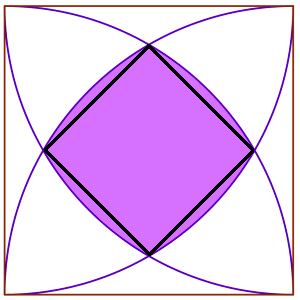#### You may also like### Baby Circle

A small circle fits between two touching circles so that all three circles touch each other and have a common tangent? What is the exact radius of the smallest circle?### Orthogonal Circle

Given any three non intersecting circles in the plane find another circle or straight line which cuts all three circles orthogonally.### Ellipses

Here is a pattern for you to experiment with using graph drawing software. Find the equations of the graphs in the pattern.

# Curved Square

##### Age 16 to 18Challenge Level
We had some really great, clear and detailed solutions for this one. It's a shame we can only write about a few of them!

Mowada and Megan used only clever coordinate geometry and the area of a sector. It can be nice to avoid tricky integrals!

Let the bottom left corner of the whole shape be the origin. We worked out the coordinates of the top point of the curved square, call this $P$. By symmetry we know that its position on the x-axis is $\frac{1}{2}$. To work out the y-value; substitute the x-value into $x^2+y^2 =1$, which is the equation of the circle the point is on.
\begin{align}
\left(\frac{1}{2}\right)^2 + y^2 &= 1\\
y^2 &= \frac{3}{4}\\
y &=\pm\frac{\sqrt 3}{2}
\end{align}
We need the positive value $y=\frac{\sqrt 3}{2}$. So the coordinates are $(\frac{1}{2},\frac{\sqrt 3}{2})$.

A very similar method can be used to find the coordinates of the right hand point of the curved square; call it $Q$. It lies on the same circle but with y coordinate equal to a half. This point has coordinates $(\frac{\sqrt 3}{2},\frac{1}{2})$.

Great work! Another way to work out the coordinates of $Q$ quickly is by symmetry; reflect the $P$ in the line $y=x$, which is the same as swapping the coordinates around.With these points worked out; we can use Pythagoras' Theorem to find the area of the largest square inside the curved square. The largest square inside has the same corners as the curved square a side length of
\begin{equation}
\sqrt{2\left(\frac{\sqrt 3}{2}-\frac{1}{2}\right)^2}=\frac{\sqrt 3 -1}{\sqrt 2}.
\end{equation}
Then the area of the largest square in the curved square is $2-\sqrt 3$.

Well done to Brandon who found this answer. But what about the curved square? Mowada and Megan guessed the size of the angle $POQ$ made at the origin between the two points $P$ and $Q$ we've already calculated. It is indeed 30$^\circ$. This could be checked with some trigonometry if you wanted to be sure.

Since $30/360=1/12$ we have that the area of the sector $OPQ$ is $\pi/12$. Also triangles $ORP$ and $ORQ$ are identical. We calculate their area. $PR$ has length $\frac{\sqrt 3 -1}{2}$ and the 'height' to $O$ is $1/2$. The area is then $\frac{\sqrt 3 -1}{8}$. The quarter of the curved square $PQR$ is then just $\frac{\pi}{12}-\frac{\sqrt 3 -1}{4}$, so the curved square has area
\begin{equation}
\frac{\pi}{3}-\sqrt 3+1.
\end{equation}
This is brilliant deduction, and not an integral in sight. Well done! Some of you might want to practice integration however, so here is Rahul's method.

We can integrate the equation of the circle that $P$ and $Q$ lie on between $x=1/2$ and $x=\sqrt{3}/2$. From this subtract the area of the rectangle with top corners $R$ and $Q$. Using the equation of a circle we need to integrate $y=\sqrt{1-x^2}$. The substitution $x=\cos t$ so that $\frac{\mathrm{d}x}{\mathrm{d}t}=-\sin t$ will be useful.
\begin{align}
\int_{1/2}^{\sqrt 3/2}\sqrt{1-x^2}\,\mathrm{d}x &= -\int_{\pi/3}^{\pi/6}(\sin t)^2\,\mathrm{d}t\\
&= \int_{\pi/6}^{\pi/3}\frac{1}{2}\left(1-\cos(2t)\right)\,\mathrm{d}t\\
&=\left[\frac{1}{2}t-\frac{1}{4}\sin(2t)\right]_{\pi/6}^{\pi/3}\\
&=\left(\frac{\pi}{6}-\frac{\sqrt 3}{8}\right)-\left(\frac{\pi}{12}-\frac{\sqrt 3}{8}\right)\\
&=\frac{\pi}{12}
\end{align}
So subtract the area of the rectangle, which is $(\sqrt 3 -1)/4$ to get a quadrant area of
\begin{equation}
\frac{\pi}{12}-\frac{\sqrt 3 -1}{4}.
\end{equation}
Great work. This is Ben's way of doing the integration. Do you have another? All that's left is to multiply by four to get the area of the whole curved square.

\begin{equation}
\text{Area(curved square)}=\frac{\pi}{3}-\sqrt 3 +1.
\end{equation}
There are many more ways to find the answer. See if you can come up with two more! Let's compare with the straight sided square inside:

\begin{align}
\text{Area(curved square)}-\text{Area(straight square)}&=\frac{\pi}{3}-\sqrt 3 +1-2+\sqrt 3\\&=\frac{\pi}{3}-1\\&\approx 0.0472\text{ (3.s.f).}
\end{align}